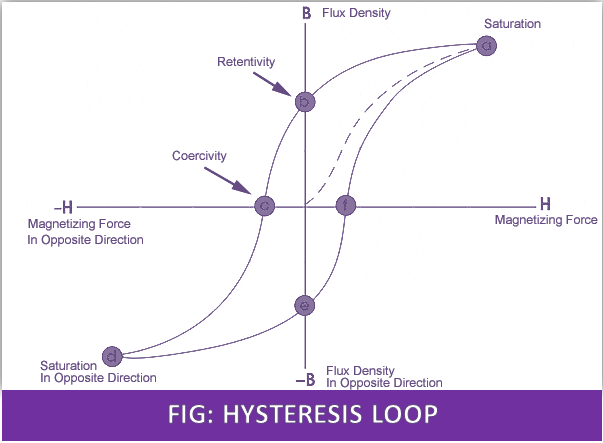# Hysteresis Loop in Magnetism

## Hysteresis Loop:

It is a closed-loop of curves obtained by plotting magnetizing force (H) against the flux density (B) for a magnetic material when the magnetizing force is taken through a complete cycle of increasing and decreasing values. The area of the loop is proportional to the magnetic hysteresis loss.## Hysteresis Loss:

The hysteresis loss in a magnetic circuit is the energy lost to overcome the molecular magnetic friction produced for the magnetizing and demagnetizing of the iron core repeatedly some heat and dissipated into the nature as a loss,

Wh = n.Bm1.6.f.v watt.
Where n= Steinmetz co-efficient, it depends upon the material.
Bm = maxm flux density, f = frequency, v=volume of iron material.

Note: Hysteresis Loss can be reduced by choosing a suitable alloy of iron. By using silicon steel the hysteresis loss can be reduced greatly.

### Magnetic Hysteresis:

It is an important property of the magnetic substance by virtue of which the magnetism developed (flux density) lags behind the magnetizing force (H) producing it. The magnetic hysteresis causes wastage of electrical energy in the form of heat.